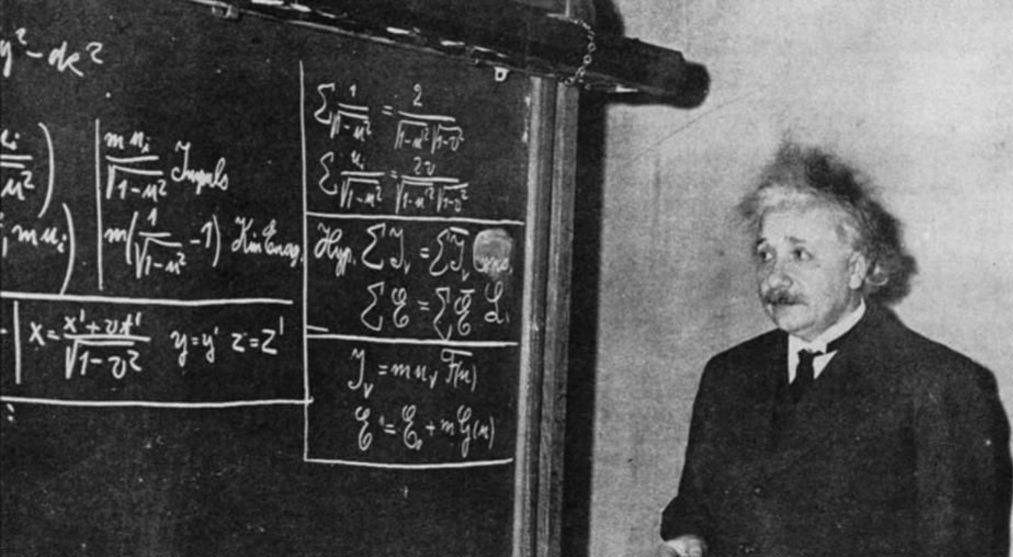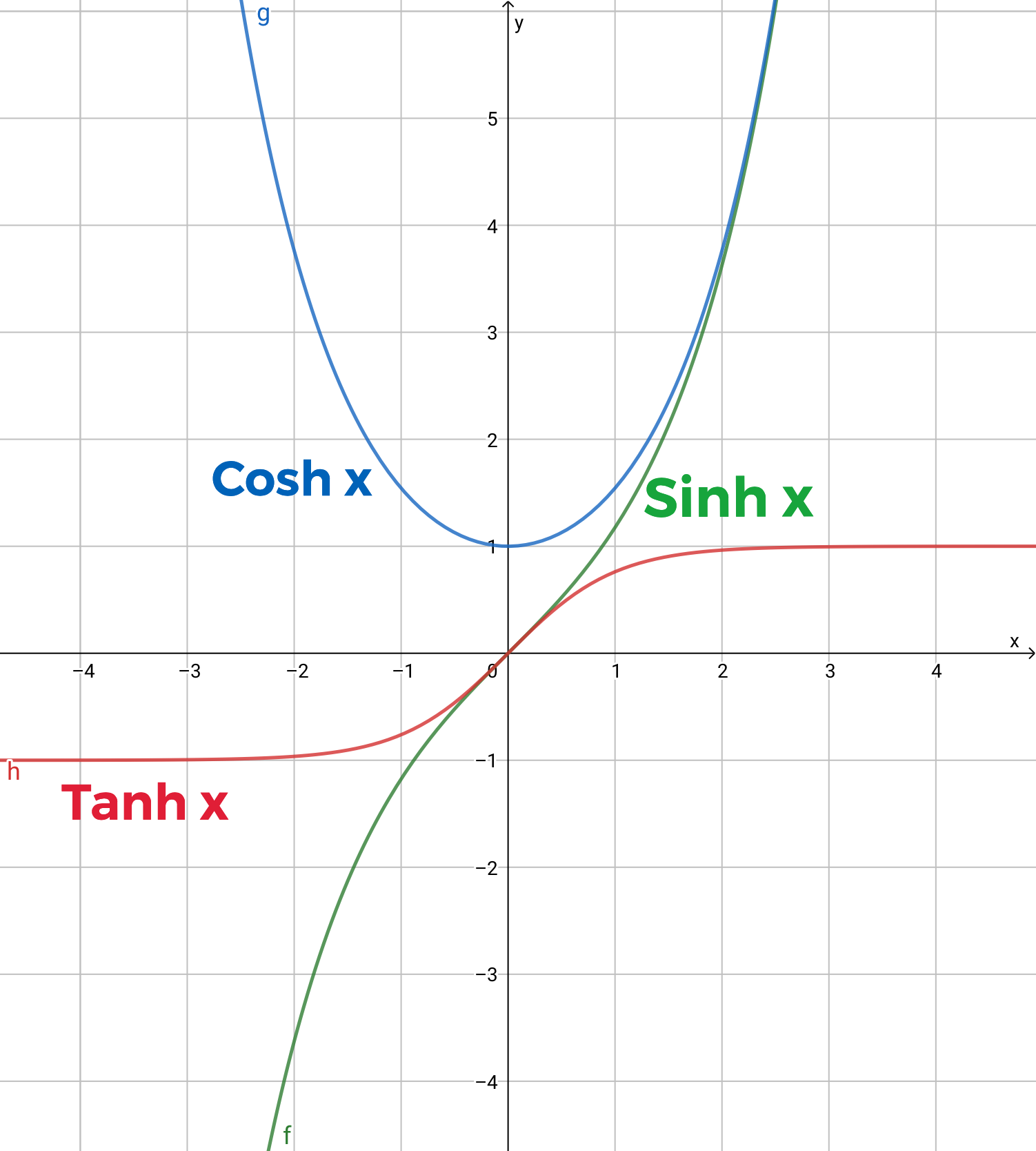• 光速不变
• 狭义相对性原理

# 一点数学知识

## 双曲函数

$$\sinh x = \frac{e^x - e^{-x}}{2},\quad \cosh x = \frac{e^x + e^{-x}}{2}$$• $\cosh^2 x - \sinh^2 x = 1$;
• $\sinh’ x = \cosh’ x$;
• $\cosh’ x = \sinh x$.

## 隐函数的微分法

$$\frac{dy}{dx} = \frac{dy(t(x))}{dx} = \frac{dy}{dt}\frac{dt}{dx}=\frac{dy}{dt}/\frac{dx}{dt}.$$

## 线性变换

\begin{equation}
\label{eq:xxbh}
\begin{cases}
\widetilde{x} = \alpha x + \beta t \\
\widetilde{t} = \gamma x + \delta t
\end{cases}
\end{equation}

\begin{equation}
\label{eq:xxbhjz}
\begin{pmatrix}
\widetilde{x}\\
\widetilde{t}
\end{pmatrix} = \begin{pmatrix}
\alpha & \beta \\
\gamma & \delta
\end{pmatrix}\begin{pmatrix}
x \\
t
\end{pmatrix}.
\end{equation}

# 线性变换下的速度公式

$$\overrightarrow{v} = \frac{d\overrightarrow{x}}{dt},\quad \widetilde{v} = \frac{d\widetilde{x}}{d\widetilde{t}}.$$

\begin{equation}
\widetilde{v} = \frac{d\widetilde{x}}{dt}/\frac{d\widetilde{t}}{dt} = \frac{\alpha\frac{d\overrightarrow{x}}{dt} + \beta}{\gamma\frac{d\overrightarrow{x}}{dt} + \delta} = \frac{\alpha\overrightarrow{v} + \beta}{\gamma\overrightarrow{v} + \delta}.
\end{equation}

# 洛伦兹变换的导出

$$\overrightarrow{x}^2-c^2 t^2 = \widetilde{x}^2-c^2 \widetilde{t}^2.$$
(这里取平方, 是为了将矢量运算变为数量的运算, 也便于导出下面的方程组.)

\begin{cases}
\alpha^2 - c^2\gamma^2 = 1\\
\alpha\beta-c^2\gamma\delta = 0\\
\beta^2 - c^2\delta^2 = -c^2
\end{cases}

\begin{equation}
\label{eq:llz1}
\begin{pmatrix}
\alpha & \beta\\
\gamma & \delta
\end{pmatrix}=\begin{pmatrix}
\cosh\theta & c\sinh\theta\\
\frac{1}{c}\sinh\theta & \cosh\theta
\end{pmatrix}
\end{equation}

$$\tanh \theta = -\frac{\overrightarrow{v_0}}{c}.$$

$$\cosh \theta = \frac{1}{\sqrt{1-\tanh^2 \theta}} = \frac{1}{\sqrt{1-\frac{v_0^2}{c}}}.$$

\begin{equation}\label{eq:llz2}
\begin{pmatrix}
\widetilde{x}\\
\widetilde{t}
\end{pmatrix} = \begin{pmatrix}
\frac{\overrightarrow{x} -\overrightarrow{v_0} t}{\sqrt{1-\frac{v_0^2}{c}}}\\
\frac{t-\frac{v_0^2}{c}x}{\sqrt{1-\frac{v_0^2}{c}}}
\end{pmatrix}
\end{equation}

\begin{equation}
\begin{pmatrix}
\widetilde{x}\\
\widetilde{t}
\end{pmatrix} = \begin{pmatrix}
\overrightarrow{x} -\overrightarrow{v_0} t\\
t
\end{pmatrix},
\end{equation}

Zengfk

2019-08-11

2021-03-09